## At an air show a jet flies directly toward the stands at a speed of 1230 km/h, emitting a frequency of 3140 Hz, on a day when the speed of s

Question

At an air show a jet flies directly toward the stands at a speed of 1230 km/h, emitting a frequency of 3140 Hz, on a day when the speed of sound is 342 m/s.

a. What frequency (in Hz) is received by the observers?
b. What frequency do they receive as the plane flies directly away from them?

in progress 0
6 months 2021-07-29T23:46:16+00:00 2 Answers 17 views 0

## Answers ( )

1. Explanation:

Given that,

Speed of the jet,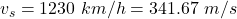Frequency of the sound produced by jet, f = 3140 Hz

Speed of sound in air, v = 342 m/s

(a) The formula of the observed frequency is given in terms of Doppler’s effect as :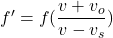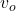is the speed of observer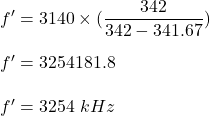(b) Let f’ is the frequency received as the plane flies directly away from them. So,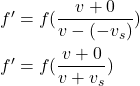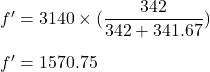Hence, this is the required solution.

a.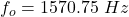b.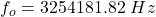which won’t be audible to the humans as the audible capacity of the human ear is from 20 Hz to 20,000 Hz.

Explanation:

Given:

• speed of jet,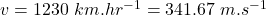• speed of sound in air,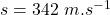• frequency of sound,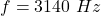a.

Since the sound source is moving therefore we can determine the observed frequency by the Doppler’s effect given as: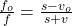where: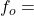observed frequency when the jet plane approaches the observers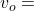observer’s velocity = 0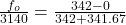b.

When the plane flies away from the observers then we take its velocity as negative.where:observed frequency when the jet plane flies away from the observersobserver’s velocity = 0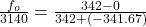which won’t be audible to the humans as the audible capacity of the human ear is from 20 Hz to 20,000 Hz.